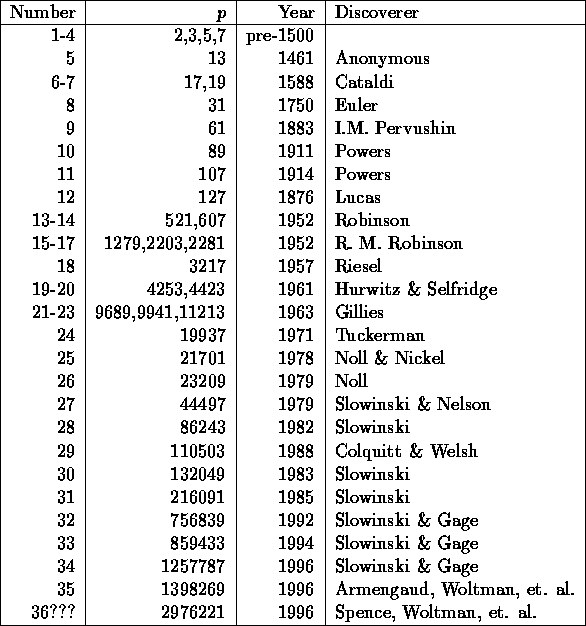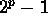Next: Formulae to compute prime Up: Prime Numbers Previous: List of record numbers

## What is the current status on Mersenne primes?

The following Mersenne primes are known.The way to determine ifis prime is to use the Lucas-Lehmer test:

```      Lucas_Lehmer_Test(p):
u := 4
for i from 3 to p do
u := u^2-2 mod 2^p-1
od
if u == 0 then
2^p-1 is prime
else
2^p-1 is composite
fi```

All exponents less than 1,481,800 have now been tested at least once.

References

An introduction to the theory of numbers. G.H. Hardy, E.M. Wright. Fifth edition, 1979, Oxford.

Alex Lopez-Ortiz
Mon Feb 23 16:26:48 EST 1998